##### Pre-Calculus: 1001 Practice Problems For Dummies (+ Free Online Practice)Polynomial functions have graphs that are smooth curves. They go from negative infinity to positive infinity in a nice, flowing fashion with no abrupt changes of direction. Pieces of polynomial functions are helpful when modeling physical situations, such as the height of a rocket shot in the air or the time a person takes to swim a lap depending on his or her age.

Most of the focus on polynomial functions is in determining when the function changes from negative values to positive values or vice versa. Also of interest is when the curve hits a relatively high point or relatively low point. Some good algebra techniques go a long way toward studying these characteristics of polynomial functions.

You’ll work with polynomial functions in the following ways:

• Rewriting quadratic equations by completing the square

• Factoring polynomials by using grouping

• Looking for rational roots of polynomials by using the rational root theorem

• Counting real roots with Descartes’s rule of signs

• Using synthetic division to quickly compute factors

• Writing equations of polynomials given roots and other information

• Graphing polynomials by using end-behavior and the factored form

Don’t let common mistakes trip you up; keep in mind that when working with polynomial functions, your challenges will include

• Watching the order of operations when using the quadratic formula

• Adding to both sides when completing the square

• Remembering to insert zeros for missing terms when using synthetic division

• Recognizing the effect of imaginary roots on the graph of a polynomial

## Practice problems

1. Find the real roots (x-intercepts) of the polynomial by using factoring by grouping.

3x3 + 2x2 – 3x – 2 = 0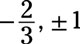First, factor by grouping. Break up the polynomial into sets of two and then find the greatest common factor of each set and factor it out. Finally, factor again.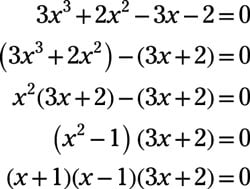Next, set each factor equal to zero and solve for x to find the x-intercepts: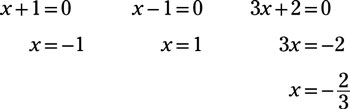2. Write an equation for the given polynomial graph.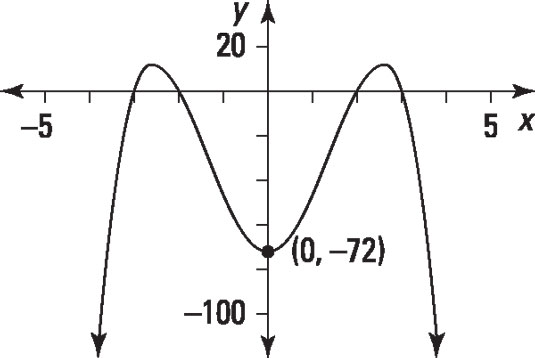Credit: Illustration by Thomson Digital

Answer: f(x) = –2x4 + 26x2 – 72

The graph crosses the x-axis at x = –3, x = –2, x = 2, and x = 3, so the function is given by

f(x) = a(x + 3)(x +2)(x –2)(x –3)

where a is a constant. The y-intercept is (0, –72), so find a by plugging in these values and solving: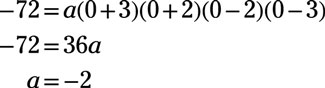Therefore, the function is f(x) = –2(x +3)(x + 2)(x –2)(x – 3) = –2x4 + 26x2 –72